2

The Fundamentals

The difficulty of learning ML has sometimes been exaggerated. There are some new rules to learn and some new habits to acquire. But most ML programmers would probably agree that ML is not inherently more difficult to understand than BASIC. More of a challenge to debug in many cases, but it's not worlds beyond BASIC in complexity. In fact, many of the first home computerists in the 1970's learned ML before they learned BASIC. This is because an average version of the BASIC language used in microcomputers takes up around 12,000 bytes of memory, and early personal computers (KIM, AIM, etc.) were severely restricted by containing only a small amount of available memory. These early machines were unable to offer BASIC, so everyone programmed in ML.

Interestingly, some of these pioneers reportedly found BASIC to be just as difficult to grasp as ML. In both cases, the problem seems to be that the rules of a new language simply are "obscure" until you know them. In general, though, learning either language probably requires roughly the same amount of effort.

The first thing to learn about ML is that it reflects the construction of computers. It most often uses a number system (hexadecimal) which is not based on ten. You will find a table in Appendix E which makes it easy to look up hex, decimal, or binary numbers.

We count by tens because it is a familiar (though arbitrary) grouping for us. Humans have ten fingers. If we had eleven fingers, the odds are that we would be counting by elevens.

What's a Natural Number?

Computers count in groups of twos. It is a fact of electronics that the easiest way to store and manipulate information is by ON-OFF states. A light bulb is either on or off. This is a two-group, it's binary, and so the powers of two become the natural groupings for electronic counters. 2, 4, 8, 16, 32, 64, 128, 256. Finger counters (us) have been using tens so long that we have come to think of ten as natural, like thunder in April. Tens isn't natural at all. What's more, twos is a more efficient way to count.

To see how the powers of two relate to computers, we can run a short BASIC program which will give us some of these powers. Powers of a number are the number multiplied by itself. Two to the power of two ( 22 ) means 2 times 2 (4). Two to the power of three ( 23 ) means 2 times 2 times 2 (8).

10 FOR I=0 to16
20 PRINT 2 ^ I
30 NEXT I

ML programming can be done in decimal (based on ten-groupings), but usually is not. Most ML programming involves hex numbers. This means groups of 16 rather than 10.

Why not just program in the familiar decimal numbers (as BASIC does)? Because 16 is one of the powers of two. It is a convenient grouping (or base) for ML because it organizes numbers the way the computer does. For example, all computers work, at the most elementary level, with bits. A bit is the smallest piece of information possible: something is either on or off, yes or no, plus or minus, true or false. This two-state condition (binary) can be remembered by a computer's smallest single memory cell. This single cell is called a bit. The computer can turn each bit "on" or "off" as if it were a light bulb or a flag raised or lowered.

It's interesting that the word bit is frequently explained as a shortening of the phrase BInary digiT. In fact, the word bit goes back several centuries. There was a coin which was soft enough to be cut with a knife into eight pieces. Hence, pieces of eight. A single piece of this coin was called a bit and, as with computer memories, it meant that you couldn't slice it any further. We still use the word bit today as in the phrase two bits, meaning 25 cents.

Whatever it's called, the bit is a small, essential aspect of computing. Imagine that we wanted to remember the result of a subtraction. When two numbers are subtracted, they are actually being compared with each other. The result of the subtraction tells us which number is the larger or if they are equal. ML has an instruction, like a command in BASIC, which compares two numbers by subtraction. It is called CMP (for compare). This instruction sets "flags" in the CPU (Central Processing Unit), and one of the flags always remembers whether or not the result of the most recent action taken by the computer was a zero. We'll go into this again later. What we need to realize now is that each flag – like the flag on a mailbox – has two possible conditions: up or down. In other words, this information (zero result or not-zero) is binary and can be stored within a single bit. Each of the flags is a bit. Together they make up one byte. That byte is called the Status Register.

Byte Assignments

Our computers group these bits into units of eight, called bytes. This relationship between bits and bytes is easy to remember if you think of a bit as one of the "pieces of eight." Eight is a power of two also (two, to the third power). Eight is a convenient number of bits to work with as a group because we can count from zero to 255 using only eight bits.

This gives us enough room to assign all 26 letters of the alphabet (and the uppercase letters and punctuation marks, etc.) so that each printed character will have its particular number. The letter "A" (uppercase) has been assigned the number 65. "B" is 66, and so on. Throughout this book, examples will follow the ASCII code for letters of the alphabet. Most microcomputers, however, do not adhere strictly to the ASCII code. If you get unexpected results when trying the example programs, check your BASIC manual to see if POKEing to the screen RAM uses a different code than ASCII. If that is the case, substitute your screen POKE code for the values given in the examples.

These "assignments" form the convention called the ASCII code by which computers worldwide can communicate with each other. Text can be sent via modems and telephone lines and arrive meaning the same thing to a different computer. It's important to visualize each byte, then, as being eight bits ganged together and able to represent 256 different things. As you might have guessed, 256 is a power of two also (two, to the power of eight).

So, these groupings of eight, these bytes, are a key aspect of computing. But we also want to simplify our counting from 0 to 255. We want the numbers to line up in a column on the screen or on paper. Obviously, the decimal number five takes up one space and the number 230 takes up three spaces.

Also, hex is easier to think about in terms of binary numbers – the on-off, single-bit way that the computer handles numbers:

 Decimal 1 2 3 4 5 6 7 8 9 10 11 12 13 14 15 16 17 Hex 01 02 03 04 05 06 07 08 09 (note new digits) ⇒ 0A 0B 0C 0D 0E 0F (note new column in the hex) ⇒ 10 11 Binary 00000001 00000010 00000011 (1 and 2) 00000100 00000101 (4 and 1) 00000110 (4 and 2) 00000111 (4+2+1) 00001000 00001001 00001010 00001011 00001100 00001101 00001110 00001111 00010000 00010001

See how hex \$10 (hex numbers are usually preceded by a dollar sign to show that they are not decimal) looks like binary? If you split a hex number into two parts, 1 and 0, and the binary (it's an eight-bit group, a byte) into two parts, 0001 and 0000 – you can see the relationship.

The Rationale For Hex Numbers

ML programmers often handle numbers as hexadecimal digits, meaning groups of sixteen instead of ten. It is usually just called hex. You should read over the instructions to the Simple Assembler and remember that you can choose between working in hex or decimal with that assembler. You can know right from the start if you're working with hex or decimal, so the dollar sign isn't used with the Simple Assembler.

DECIMAL    0 1 2 3 4 5 6 7 8 9    then you start over with 10

HEX 00 01 02 03 04 05 06 07 08 09 0A 0B 0C 0D 0E 0F    then you start over with 10

Program 2-1. Microsoft Hex-Decimal Converter.

1 HE\$="0123456789ABCDEF"
4 PRINT"{03 DOWN}{03 RIGHT}1-INPUT HEX & GET DECIMAL BACK.
5 REM NEW LINE HERE
6 PRINT"{02 DOWN}    2-INPUT DECIMAL TO GET HEX BACK.
7 GETK:IFK=0THEN7
9 PRINT"{CLEAR}":ON K-GOTO200,400
100 H\$="":FORM=3TO0STEP-1:N%=DE/(16^M):DE=DE-N%*16^M:H\$=H\$+MID\$(HE\$,N%+1,1):NEXT
101 RETURN
102 D=0:Q=3:FORM=1TO4:FORW=0TO15:IFMID\$(H\$,M,1)=MID\$(HE\$,W+1,1)THEN104
103 NEXTW
104 D1=W*(16^(Q)):D=D+D1:Q=Q-1:NEXTM
105 DE=INT(D):RETURN
200 INPUT"{02 DOWN}HEX";H\$:GOSUB102:PRINTSPC(11)"{UP}= {REV}"DE"{LEFT} "
210 GOTO200
400 INPUT"{02 DOWN}DECIMAL";DE:GOSUB100:PRINTSPC(14)"{UP}= {REV} "H\$" "
410 GOTO400

Program 2-2. Atari Hex-Decimal Converter.

100 DIM H\$(23),N\$(9):OPEN#1,4,0,"K:"
130 GRAPHICS 0
150 PRINT "1- INPUT HEX AND GET DECIMAL BACK."
160 PRINT "2- INPUT DECIMAL AND GET HEX BACK."
170 PRINT:PRINT "==>";:GET#1,K
180 IF K<49 OR K>50 THEN 170
190 PRINT CHR\$(K):ON K-48 GOTO 300,400
300 H\$="@ABCDEFGHI!!!!!!!JKLMNO"
310 PRINT "HEX";:INPUT N\$:N=0
320 FOR I=1 TO LEN(N\$)
330 N=N*16+ASC(H\$(ASC(N\$(I))-47))-64:NEXT I
340 PRINT "\$";N\$;"=";N:PRINT:PRINT:GOTO 140
400 H\$="0123456789ABCDEF"
410 PRINT "DECIMAL";:INPUT N:M=4096
420 PRINT N;"=\$";
430 FOR I=1 TO 4:J=INT(N/M)
440 PRINT H\$(J+1,J+1);:N=N-M*J:M=M/16
450 NEXT I:PRINT:PRINT:GOTO 140

The first thing to notice is that instead of the familiar decimal symbol 10, hex uses the letter "A" because this is where decimal numbers run out of symbols and start over again with a one and a zero. Zero always reappears at the start of each new grouping in any number system: 0, 10, 20, etc. The same thing happens with the groupings in hex: 0, 10, 20, 30, etc. The difference is that, in hex, the 1 in the "tens" column equals a decimal 16. The second column is now a "sixteens" column. 11 means 17, and 21 means 33 (2 times 16 plus one). Learning hex is probably the single biggest hurdle to get over when getting to know ML. Don't be discouraged if it's not immediately clear what's going on. (It probably never will be totally clear – it is, after all, unnatural.) You might want to practice the exercises at the end of this chapter. As you work with ML, hex will gradually seem less and less alien.

To figure out a hex number, multiply the second column by 16 and add the other number to it. So, 1A would be one times 16 plus 10 (recall that A stands for ten).

Hex does seem impossibly confusing when you come upon it for the first time. It will never become second nature, but it should be at least generally understood. What is more, you can program in ML quite easily by looking up the hex numbers in the table at the end of this book. You need not memorize them beyond learning to count from 1 to 16 – learning the symbols. Be able to count from 00 up to 0F. (By convention, even the smallest hex number is listed as two digits as in 03 or 0B. The other distinguishing characteristic is that dollar sign that is usually placed in front of them: \$05 or \$0E.) It is enough to know what they look like and be able to find them when you need them.

The First 255

Also, most ML programming involves working with hex numbers only between 0 and 255. This is because a single byte (eight bits) can hold no number larger than 255. Manipulating numbers larger than 255 is of no real importance in ML programming until you are ready to work with more advanced ML programs. This comes later in the book. For example, all 6502 ML instructions are coded into one byte, all the "flags" are held in one byte, and many "addressing modes" use one byte to hold their argument.

To learn all we need know about hex for now, we can try some problems and look at some ML code to see how hex is used in the majority of ML work. But first, let's take an imaginary flight over computer memory. Let's get a visual sense of what bits and bytes and the inner workings of the computer's RAM look like.

The City Of Bytes

Imagine a city with a single long row of houses. It's night. Each house has a peculiar Christmas display: on the roof is a line of eight lights. The houses represent bytes; each light is a single bit. (See Figure 2-1.) If we fly over the city of bytes, at first we see only darkness. Each byte contains nothing (zero), so all eight of its bulbs are off. (On the horizon we can see a glow, however, because the computer has memory up there, called ROM memory, which is very active and contains built-in programs.) But we are down in RAM, our free user-memory, and there are no programs now in RAM, so every house is dark. Let's observe what happens to an individual byte when different numbers are stored there; we can randomly choose byte 1504. We hover over that house to see what information is "contained" in the light display. (See Figure 2-2.)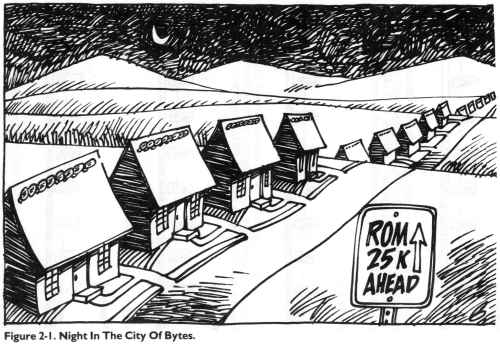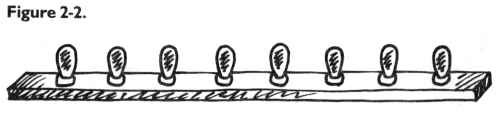Like all the rest, this byte is dark. Each bulb is off. Observing this, we know that the byte here is "holding" or representing a zero. If someone at the computer types in POKE 1504,1 – suddenly the rightmost light bulb goes on and the byte holds a one instead of a zero: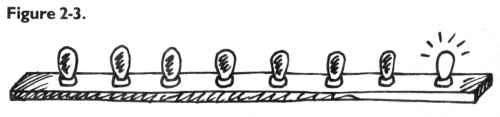This rightmost bulb is in the 1's column (just as it would be in our usual way of counting by tens, our familiar decimal system). But the next bulb is in a 2's column, so POKE 1504,2 would be: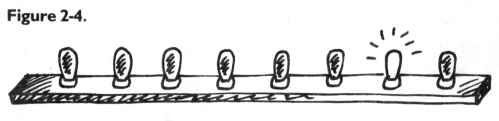And three would be one and two: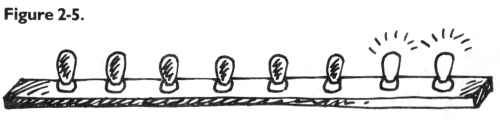In this way – by checking which bits are turned on and then adding them together – the computer can look at a byte and know what number is there. Each light bulb, each bit, is in its own special position in the row of eight and has a value twice the value of the one just before it: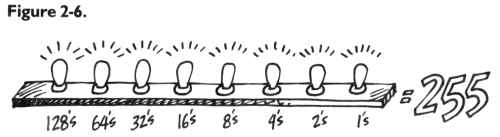Eight bits together make a byte. A byte can "hold" a number from 0 through 255 decimal. We can think of bytes, though, in any number system we wish – in hex, decimal, or binary. The computer uses binary, so it's useful to be able to visualize it. Hex has its uses in ML programming. And decimal is familiar. But a number is still a number, no matter what we call it. After all, five trees are going to be five trees whether you symbolize them by 5, \$05, or 00000101.

A Binary Quiz

BASIC doesn't understand numbers expressed in hex or binary. The Simple Assembler contains two subroutines to translate a number from decimal to hex or vice versa. You might want to take a look at how it's done as a way of getting a better feel for these different numbers systems. The subroutines are located at lines 4000 and 5000. Binary, for humans, is very visual. It forms patterns out of zeros and ones. The following program will let you quiz yourself on these patterns.

Here is a game, for all computers, which will show you a byte as it looks in binary. You then try to give the number in decimal:

Program 2-3. Binary Quiz for All Computers.

100 REM BINARY QUIZ
110 C1=20:C0=111: REM FOR ATARI ONLY
120 Cl=88:C0=79:  REM FOR APPLE ONLY
130 Cl=209:C0=215:REM FOR COMMODORE ONLY
140 X=INT(256*RND(1)):D=X:P=128
150 PRINT CHR\$(125);: REM ATARI ONLY
160 PRINT CHR\$(147);: REM COMMODORE ONLY
170 HOME: REM APPLE ONLY
180 FOR I=1 TO 8
190 IF INT(D/P)=1 THEN PRINT CHR\$(C1);:D=D-P:GOTO 210
200 PRINT CHR\$(C0);
210 P=P/2:NEXT I:PRINT
220 PRINT "WHAT IS THIS IN DECIMAL?"
230 INPUT Q:IF Q=X THEN PRINT "CORRECT":GOTO 250
240 PRINT "SORRY, IT WAS ";X
250 FOR T=1 TO 1000:NEXT T
260 GOTO 140

This program will print out the entire table of binary numbers from 0 to 255:

Note from AtariArchives editors: This program will work with any version of Microsoft BASIC including modern versions such as QuickBasic and QBasic. It will not run properly under Atari BASIC. The reason for this is that the "AND" operators in lines 140 and 160 function as bitwise operators rather than Boolean operators. Atari BASIC does not implement bitwise operators.

Program 2-4.

100 REM Complete Binary Table
110 L=8:B=2:C=1
120 FOR X=0 TO 255:PRINT X;": ";
140 IF X AND C THEN K(C)=49:GOTO 160
150 K(C)=48
160 C=C+1:IF B AND X THEN K(C)=49:GOTO 180
170 K(C)=48
180 B=B*2:IF C>8 THEN 200
190 GOTO 160
200 FOR I=0 TO 7:PRINT STR\$(K(L)-48);:L=L-1
210 NEXT
220 C=0:PRINT
260 L=8:B=2:C=1:NEXT X

Examples And Practice

Here are several ordinary decimal numbers. Try to work out the hex equivalent:

 1. 2. 3. 4. 5. 6. 7. 8. 9. 10. 10 15 5 16 17 32 128 129 255 254 ______ ______ ______ ______ ______ ______ ______ ______ ______ ______

We are not making an issue of learning hex or binary. If you needed to look up the answers in the table at the end of the book, fine. As you work with ML, you will familiarize yourself with some of the common hex numbers. You can write most ML programs without needing to worry about binary. For now, we only want to be able to recognize what hex is. There are even some pocket "programmer" calculators which change decimal to hex for you and vice versa. Another way to go about "hexing" is to use a BASIC program which does the translation. A problem with BASIC is that you will be working in ML and your computer will be tied up. It is often inconvenient to crank up a BASIC program each time you need to work out a hex number. However, the Simple Assembler will do the translations for you any time you need them.

One other reason that we are not stressing hex too much is that ML is generally not programmed without the help of an assembler. The Simple Assembler provided in this book will handle most of your input automatically. It allows you to choose whether you prefer to program in hex or decimal. You make this decision by changing line 10 before starting to assemble. After that, you can put in hex or decimal without worrying that there will be any confusion about your intentions.

This little BASIC program is good for practicing hex, but also shows how to change a small part and make it work for two-byte hex numbers. It will take decimal in and give back the correct hex. It is designed for Microsoft BASIC computers, so it will not work on the Atari.

10 H\$="0123456789ABCDEF"
20 PRINT "ENTER DECIMAL NUMBER";:INPUT X
30 IF X > 255 GOTO 20: REM NO NUMBERS BIGGER THAN 255 ALLOWED
40 FOR I=1 TO 0 STEP -1
50 N%=X/(16^I):X=X-N%*16^I
60 HE\$=HE\$+MID\$(H\$,N%+1,1)
70 NEXT
80 PRINT HE\$
90 GOTO 20

For larger hex numbers (up to two, to the power of 16 – which is 65536), we can just change the above program. Eliminate line 30 and change line 40 to: FOR I=3 TO 0 STEP -1. This will give us four-place hex numbers (used only as addresses) but which will also become recognizable after some ML practice.

65535 is an interesting number because it represents the limit of our computers' memories. In special cases, with additional hardware, memory can be expanded beyond this. But this is the normal upper limit because the 6502 chip is designed to be able to address (put bytes in or take them out of memory cells) up to \$FFFF.

Ganging Two Bytes Together To Form An Address

The 6502 often sets up an address by attaching two bytes together and looking at them as if they formed a unit. An address is most commonly a two-byte number. \$FFFF (65535) is the largest number that two bytes can represent, and \$FF (255) is the most that one byte can hold. Three-byte addressing is not possible for the 6502 chip. "Machine language" means programming which is understood directly by the 6502 chip itself. There are other CPU (Central Processing Unit) chips, but the 6502 is the CPU for VIC, Apple, 64, PET/CBM, and Atari. It's the one covered in this book.

Before getting into an in-depth look at "monitors," those bridges between you and your machine's language – we should first learn how to read ML program listings. You've probably seen them often enough in magazines. Usually, these commented, labeled, but very strange-looking programs are called source code. They can be examined and translated by an assembler program into an ML program. When you have an assembler program run through source code, it looks at the key words and numbers and then POKEs a series of numbers into the computer. This series is then called the object code.

Source programs contain a great deal of information which is of interest to the programmer. The computer only needs a list of numbers which it can execute in order. But for most people, lists of numbers are only slightly more understandable than Morse code. The solution is to replace numbers with words. The primary job of an assembler is to recognize an ML instruction. These instructions are called mnemonics, which means "memory aids." They are like BASIC words, except that they are always three letters long.

If you type the mnemonic JMP, the assembler POKEs a 76 into RAM memory. It's easier to remember JMP than 76. The 76 is the number that clues the computer that it's supposed to perform a JMP. The 76 is called an opcode, for "operation code." The three-letter words we use in ML programming, the mnemonics, were designed to sound like what they do. JMP does a JUMP (like a GOTO in BASIC). Some deluxe assemblers also let you use labels instead of numbers – as long as you define your labels at the start of the source code. These labels can refer to individual memory locations, special values like the score in a game, or entire subroutines.

Four Ways To List A Program

Labeled, commented source code listings are the most elaborate kind of ML program representation. There are also three other kinds of ML listings. We can use a simple addition example program to show how it looks when represented in each of the four ML program listing styles. The first two styles are simply ways for you to type a program into the computer. The last two styles show you what to type in, but also illustrate what is going on in the ML program. First let's look at the most elementary kind of ML found in books and magazines: the BASIC loader.

10 FOR ADDRESS = 4096 TO 4103
50 DATA 169,2,105,5,141,160,15,96

This is a series of decimal numbers in DATA statements which is POKEd into memory starting at decimal address 4096. When these numbers arrive in RAM, they form a little routine which puts the number 2 into the accumulator – a special location in the computer that we'll get to later - and then adds 5. The result of the addition is then moved from the accumulator to decimal address 4000. If you try this program out, you can SYS 4096 to execute ML program and then ? PEEK (4000) and you'll see the answer: seven. BASIC loaders are convenient because the user doesn't need to know how to enter ML programs. The loader POKEs them in and all the user has to do is SYS or USR or CALL to the right address and the ML transfers control back to BASIC when its job is done.

Getting even closer to the machine level is the second way you might see ML printed in books or magazines: the hex dump. On some computers (PET, Apple) there is a special "monitor" program in ROM which lets you list memory addresses and their contents as hex numbers. More than that, you can usually type over the existing values on the screen and change them. That's what a hex dump listing is for. You copy it into your computer's RAM by using your computer's monitor. How you enter the monitor mode differs on each computer and we'll get to monitors in the next chapter.

The hex dump, like the BASIC loader, tells you nothing about the functions or strategies employed within an ML program. Here's the hex dump version of the same 2+5 addition program:

Program 2-7.

1000 A9 02 69 05 8D A0 0F 60

The third type of listing is called a disassembly. It's the opposite of an assembly because another program called a disassembler takes machine language (the series of numbers, the opcodes in the computer's memory) and translates it into the words, the mnemonics, which ML programmers use. The instruction you use when you want to load the accumulator is called LDA, and you can store what's in the accumulator by using an STA. We'll get to them later. In this version of our example addition routine, it's a bit clearer what's going on and how the program works. Notice that on the left we have the hex numbers and, on the right, the translation into ML instructions. ADC means ADd with Carry and RTS means ReTurn from Subroutine.

Program 2-8.

1000 A9 02    LDA #\$02
1004 8D A0 0F STA \$0FA0
1007 60       RTS

The Deluxe Version

Finally we come to that full, luxurious, commented, labeled, deluxe source code we spoke of earlier. It includes the hex dump and the disassembly, but it also has labels and comments and line numbers added, to further clarify the purposes of things. Note that the numbers are all in hex. On the far left are the memory addresses where this routine is located. Next to them are the hex numbers of the instructions. (So far, it resembles the traditional hex dump.) Then come line numbers which can be used the way BASIC line numbers are: deleted, inserted, and so on. Next are the disassembled translations of the hex, but you can replace numbers with labels (see Program 2-10). You could still use numbers, but if you've defined the labels early on, they can serve as a useful reminder of what the numbers represent. Last, following the semicolons, are the comments. They are the same as REM statements. (See Programs 2-9 and 2-10.)

Program 2-9. A Full Assembly Listing.      -------------Source Code -----------

 Memory Address Object Code Line Number Disassembly Comments 0005 .BA \$1000 ; START ADDR \$1000 (4096) 1000- A9 02 0100 LDA #\$02 ; LOAD A WITH 2 1002- 69 05 0110 ADC #\$05 ; ADD 5 1004- 8D A0 0F 0120 STA \$0FA0 ; STORE AT DECIMAL 4000 1007- 60 0130 RTS ; RETURN 0140 .EN ; END OF ASSEMBLY

Program 2-10. Labelled Assembly.

 0005 .BA \$1000 ; START ADDR \$1000 (4096) 0010 TWO .DE 2 ;DEFINE LABEL "TWO" AS 2. 0020 ADDER .DE 5 ;DEFINE "ADDER" AS A 5. 0030 STORAGE .DE \$0FA0 ;DEFINE STORAGE ADDR. 0040 ; 1000- A9 02 0100 START LDA #TWO ; LOAD A WITH 2 1002- 69 05 0110 ADC #ADDER ; ADD 5 1004- 8D A0 0F 0120 STA STORAGE ; STORE AT DECIMAL 4000 1007- 60 0130 RTS ; RETURN 0140 .EN ; END OF ASSEMBLY

--- LABEL FILE: ---
ADDER =0005    START =1000    STORAGE =0FA0
TWO =0002

Program 2-11. The Source Code By Itself.

 .BA \$1000 ; START ADDR \$1000 (4096) TWO .DE 2 ;DEFINE LABLE "TWO" AS 2. ADDER .DE 5 ;DEFINE "ADDER" AS A 5. STORAGE .DE \$0FA0 ;DEFINE STORAGE ADDR. ; START LDA #TWO ; LOAD A WITH 2 ADC #ADDER ; ADD 5 STA STORAGE ; STORE AT DECIMAL 4000 RTS ; RETURN .EN ; END OF ASSEMBLY

Program 2-11 illustrates just the source code part. The object code has not yet been generated from this source code. The code has not been assembled yet. You can save or load source code via an assembler in the same way that you can save or load programs via BASIC. When 2-11 is in the computer, you could type "ASSEMBLE" and the assembler would translate the instructions, print them on the screen, and POKE them into memory.

The Simple Assembler operates differently. It translates, prints, and POKEs after you hit RETURN on each line of code. You can save and load the object, but not the source code.

Before we get into the heart of ML programming, a study of the instruction mnemonics and the various ways of moving information around (called addressing), we should look at a major ML programming aid: the monitor. It deserves its own chapter.

ANSWERS to quiz: 0A, 0F, 05, 10, 11, 20, 80, 81, FF, FE